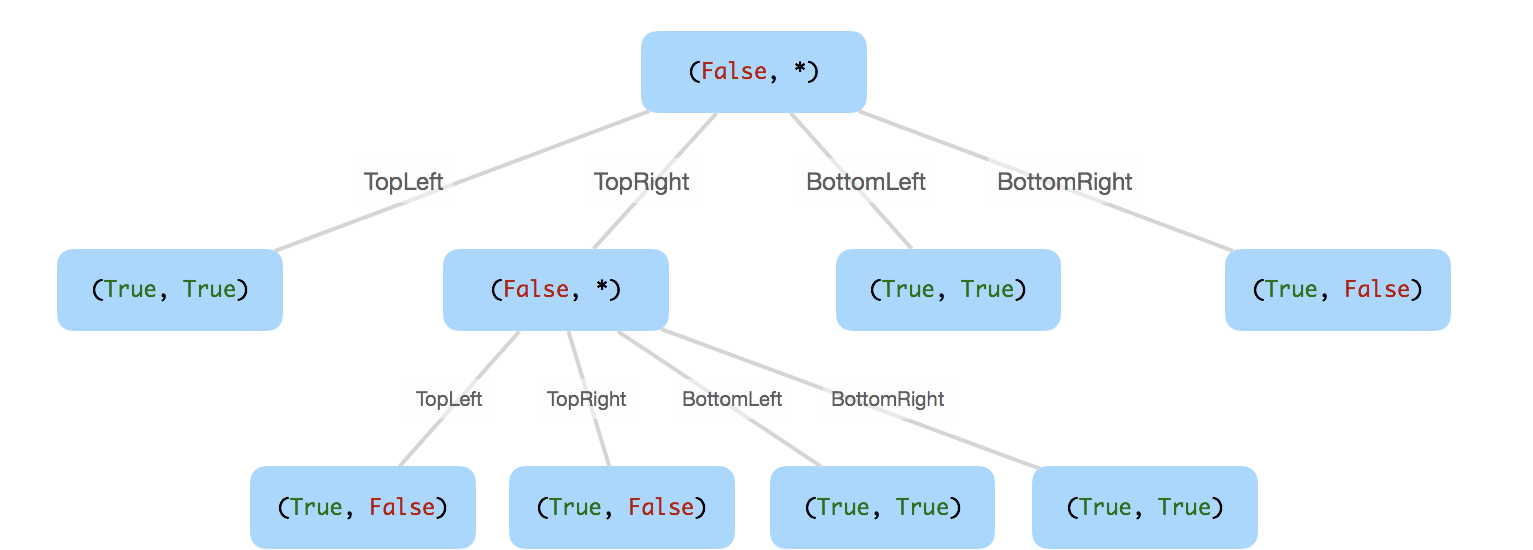Similar Problems:

We want to use quad trees to store an N x N boolean grid. Each cell in the grid can only be true or false. The root node represents the whole grid. For each node, it will be subdivided into four children nodes until the values in the region it represents are all the same.

Each node has another two boolean attributes : isLeaf and val. isLeaf is true if and only if the node is a leaf node. The val attribute for a leaf node contains the value of the region it represents.

Given the 8 x 8 grid below, we want to construct the corresponding quad tree:

It can be divided according to the definition above:

The corresponding quad tree should be as following, where each node is represented as a (isLeaf, val) pair.

For the non-leaf nodes, val can be arbitrary, so it is represented as *.Note:

1. N is less than 1000 and guaranteened to be a power of 2.
2. If you want to know more about the quad tree, you can refer to its wiki.

Github: code.dennyzhang.com

Credits To: leetcode.com

Leave me comments, if you have better ways to solve.

• Solution:
```## Blog link: https://code.dennyzhang.com/construct-quad-tree
## Basic Ideas: post-order
##
## Complexity: Time O(n*n) Space O(1)
"""
# Definition for a QuadTree node.
class Node:
def __init__(self, val, isLeaf, topLeft, topRight, bottomLeft, bottomRight):
self.val = val
self.isLeaf = isLeaf
self.topLeft = topLeft
self.topRight = topRight
self.bottomLeft = bottomLeft
self.bottomRight = bottomRight
"""
class Solution:
def construct(self, grid):
"""
:type grid: List[List[int]]
:rtype: Node
"""
def myConstruct(grid, i, j, count):
if count <= 0: return None
if count == 1: return Node(grid[i][j], True, None, None, None, None)
count2 = int(count/2)
topLeft = myConstruct(grid, i, j, count2)
topRight = myConstruct(grid, i, j+count2, count2)
bottomLeft = myConstruct(grid, i+count2, j, count2)
bottomRight = myConstruct(grid, i+count2, j+count2, count2)
if topLeft.isLeaf and topRight.isLeaf \
and bottomLeft.isLeaf and bottomRight.isLeaf \
and topLeft.val == topRight.val \
and topLeft.val == bottomLeft.val \
and topLeft.val == bottomRight.val:
return Node(topLeft.val, True, None, None, None, None)
else:
return Node(None, False, topLeft, topRight, bottomLeft, bottomRight)

return myConstruct(grid, 0, 0, len(grid))
```

Share It, If You Like It.